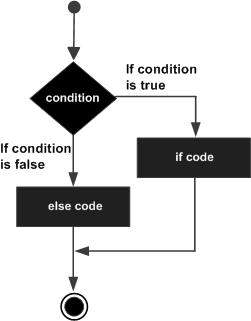# C++ if...else statement

An if statement can be followed by an optional else statement, which executes when the boolean expression is false.

## Syntax

The syntax of an if...else statement in C++ is −

```if(boolean_expression) {
// statement(s) will execute if the boolean expression is true
} else {
// statement(s) will execute if the boolean expression is false
}
```

If the boolean expression evaluates to true, then the if block of code will be executed, otherwise else block of code will be executed.

## Flow Diagram## Example

```#include <iostream>
using namespace std;

int main () {
// local variable declaration:
int a = 100;

// check the boolean condition
if( a < 20 ) {
// if condition is true then print the following
cout << "a is less than 20;" << endl;
} else {
// if condition is false then print the following
cout << "a is not less than 20;" << endl;
}
cout << "value of a is : " << a << endl;

return 0;
}
```

When the above code is compiled and executed, it produces the following result −

```a is not less than 20;
value of a is : 100
```

## if...else if...else Statement

An if statement can be followed by an optional else if...else statement, which is very usefull to test various conditions using single if...else if statement.

When using if , else if , else statements there are few points to keep in mind.

• An if can have zero or one else's and it must come after any else if's.

• An if can have zero to many else if's and they must come before the else.

• Once an else if succeeds, none of he remaining else if's or else's will be tested.

## Syntax

The syntax of an if...else if...else statement in C++ is −

```if(boolean_expression 1) {
// Executes when the boolean expression 1 is true
} else if( boolean_expression 2) {
// Executes when the boolean expression 2 is true
} else if( boolean_expression 3) {
// Executes when the boolean expression 3 is true
} else {
// executes when the none of the above condition is true.
}
```

## Example

```#include <iostream>
using namespace std;

int main () {
// local variable declaration:
int a = 100;

// check the boolean condition
if( a == 10 ) {
// if condition is true then print the following
cout << "Value of a is 10" << endl;
} else if( a == 20 ) {
// if else if condition is true
cout << "Value of a is 20" << endl;
} else if( a == 30 ) {
// if else if condition is true
cout << "Value of a is 30" << endl;
} else {
// if none of the conditions is true
cout << "Value of a is not matching" << endl;
}
cout << "Exact value of a is : " << a << endl;

return 0;
}
```

When the above code is compiled and executed, it produces the following result −

```Value of a is not matching
Exact value of a is : 100
```
cpp_decision_making.htm